/

### The Nematrian Website’s Complex Number Function Algorithms

The Nematrian website contains a suite of interactive pages and web services that permit users to manipulate complex numbers. Complex numbers are extensions to real numbers that historically owe their introduction principally to attempts to solve algebraic equations, in the first instance the equation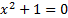.

Each complex number has two components, its real part and its imaginary part, and can thus be plotted in the so called complex plane where the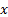-coordinate refers to the real part and the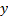-coordinate refers to the imaginary part of the complex number. Any real number can be considered to be a complex number that has a zero imaginary part. Conventionally, a complex number is written as, say,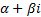, where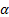is its real part, and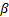is its imaginary part, andis a purely complex number defined to be the square root of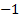that has positive imaginary part (to distinguish it from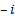which is also then a square root of). Complex numbers adhere to the following extensions to the usual rules for adding, subtracting, multiplying and dividing real numbers: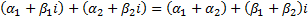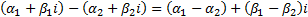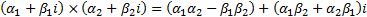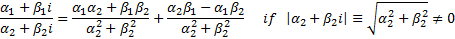Any polynomial equation of order(>0), i.e. of the form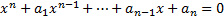(where the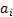are either real or complex) in general hasroots, i.e. solutions, some of which may be real and some of which may be complex (although not all of these roots are necessarily distinct). Large parts of the mathematical theory of real analysis carry over to the complex case, indeed in many instances introduction of complex number theory simplifies and streamlines these results, e.g. the theory of analytic functions.

The functions (web services) that the Nematrian makes available for manipulation of complex numbers are described further in ComplexNumbersFunctions.

To pass complex values to these web services, each number that would otherwise have been a single ‘real’ variable needs to be replaced by two numbers, the first being the real part of the complex number and the second part of the complex number. Thus, if the equivalent real function expected a single real number (typically a ‘double precision’ number using computer algorithm terminology) then the corresponding Nematrian function taking a complex number argument would expect to receive an array of two such numbers. If the equivalent real function expected an array of real numbers then the corresponding Nematrian function taking a complex number array argument would expect to receive an array of twice the size, with the first and second elements of the array being the real and imaginary parts of the corresponding first element of the real number equivalent, the third and fourth corresponding to the second element of the real number equivalent etc.

Some complex functions can be multivalued. For example,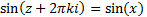for all real (or complex)and integral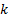. Thus a function such as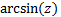, i.e. the valuefor which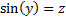, does not in general have a unique value. Instead, it is usual for complex number function algorithms to focus merely on the principal value of the number, typically taken to mean the one for which choice ofgives the smallest absolute size for the imaginary part of the answer.